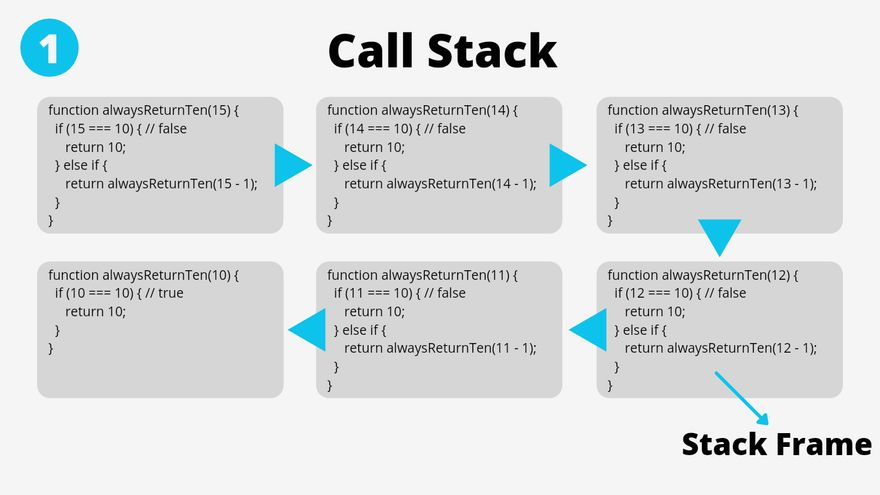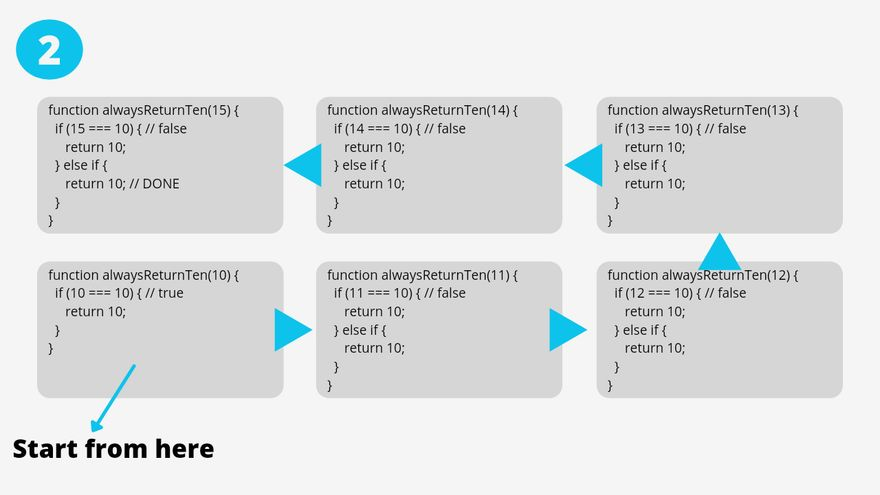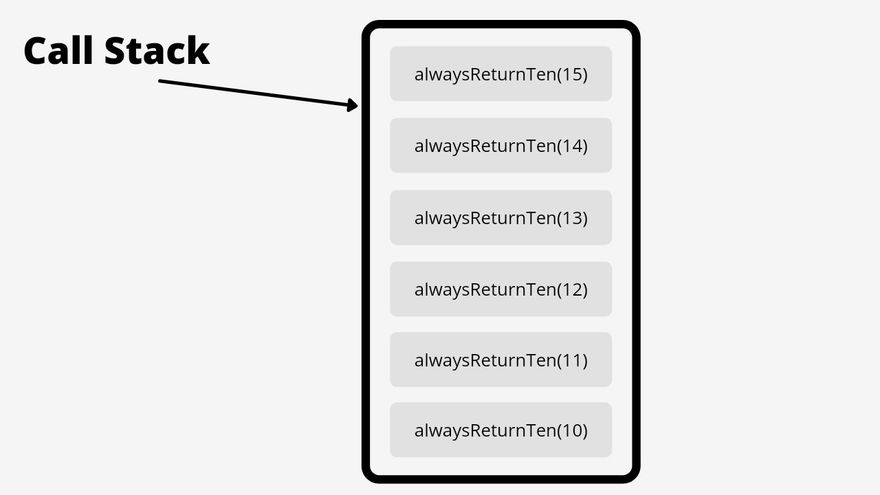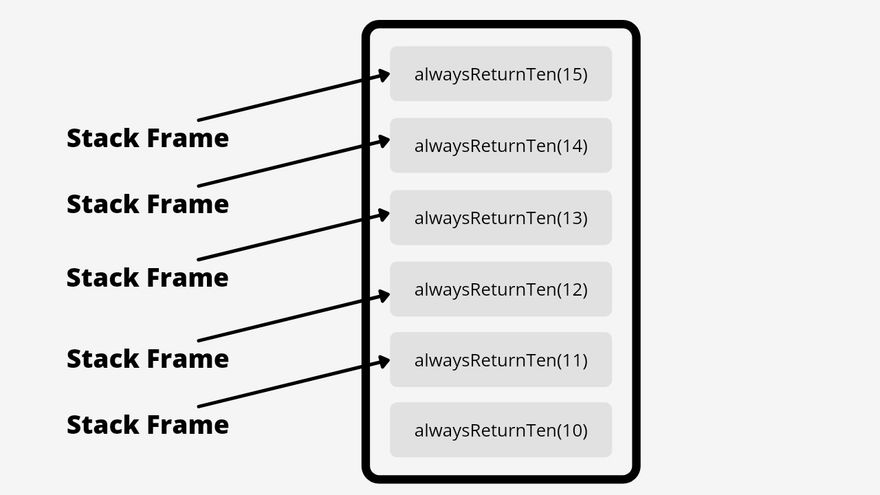## CodeNewbie Community is a community of 5,577 amazing newbies# Recursion In Programming (Simplified Version)

Recursion may seem confusing and hard to understand at first, but in this article, I'm going to explain it in a very simple and friendly way.

## What Is Recursion?Learning recursion is reminded me about SpongeBob SquarePants. Anyway, what is recursion? Recursion is simply a function that call itself until it gets the job done. Let's take a look at the dummy function that always return ten no matter how much numbers that you passed in.

``````function alwaysReturnTen(number) {
if (number === 10) {
return 10;
} else if (number > 10) {
return alwaysReturnTen(number - 1);
} else if (number < 10) {
return alwaysReturnTen(number + 1);
}
}

alwaysReturnTen(15) // return 10
``````

Don't forget to try it yourself 😉.

Let's see what's going on this function.

• So first, we passed in number 15 to the `alwaysReturnTen()` function.
• Next we check whether it is already `10` or not. Which is the base case of this function.
``````function alwaysReturnTen(15) {
if (15 === 10) { // false
return 10;
}
}
``````
• As you can see, it's not satisfied the base condition, so we move to the next block of code.
``````function alwaysReturnTen(15) {
if (15 === 10) { // false
return 10;
} else if (15 > 10) { // true
return alwaysReturnTen(15 - 1);
// which is equal to:
// alwaysReturnTen(14)
}
}
``````
• At this point we're checking, is `15` is greater than `10`? The answer is yes so we call the function again (do a recursive call) but, we passed in with a different argument.
• So now, we have to finish the next task, which is, "what `alwaysReturnTen(14)` will give us?"
• Again, we try to check, "is `14` equal to `10`?" and the answer is NO.
``````function alwaysReturnTen(14) {
if (14 === 10) { // false
return 10;
}
}
``````
• And again, we're checking, "is `14` greater than `10`?" The answer is yes so we call the function again but, we passed in with another argument, which is `14 - 1`.
``````function alwaysReturnTen(14) {
if (14 === 10) { // false
return 10;
} else if (14 > 10) { // true
return alwaysReturnTen(14 - 1);
// which is equal to:
// alwaysReturnTen(13)
}
}
``````
• The process keep repeating, until we hit the base case which is `10`.
• Once we passed in `10`, then the function will return `10`.
``````function alwaysReturnTen(11 - 1) {
if (10 === 10) {
return 10;
}
// That's it, we finally stop the
// recursive call and get 10.
}
``````
• Now, it's not done yet. We need to finish another the rest of the jobs that gets delayed.
• So, we basically swap the `alwaysReturnTen(11 - 1)` with number `10`.
``````function alwaysReturnTen(11) {
if (11 === 10) {
return 10;
} else if (11 > 10) {
return 10; // return 10
}
}
``````
• The process keep repeating until we get to the first call of the recursive function, which is `alwaysReturnTen(15)`.
• And since all recursive call function return `10`, then `alwaysReturnTen(15)` will give us `10`.

Get confused? Take a look at these images below.### Call StackThe call stack is basically where all the processes that are not finished get stored in order. You can think of it like an array that holds a bunch of stack frame.

### Stack FrameEach item inside the call stack called stack frame. As simple as that.

## Common Error in RecursionCreate a recursive function without a good base case can make your program crash and produce an error which is commonly known as StackOverflow Error.

A simple recursive function to achieve a StackOverflow Error.

``````function badFunction() {
}

// Uncaught RangeError: Maximum call
// stack size exceeded
``````

So, to see why this is happening, let's add `console.log(10)` inside the function.

``````function badFunction() {
console.log(10);
}

// Now, we get a lot of 10 in our console
// and this error 👇
// Uncaught RangeError: Maximum call
// stack size exceeded
``````

So what really happened behind the scenes was that, it keeps doing `console.log` until it reaches the maximum call stacks(memories), which basically means the computer is "surrender" to run the function.

If we analyze that function, we know that what makes recursive function is bad or I should say useless is that:

Bad stopping condition or no stopping condition.

## Further Learning

I recommend to watch this video from FreeCodeCamp about Recursion and read the articles about recursion for further learning.

I also recommend to improve that `alwaysReturnTen()` function, since if I passed in a float or decimal number like `10.5`, then it will never be resolved.

## Thanks!

Thank you everyone for reading it until this point. Hopefully this is helpful and make you understand about the basic of recursion. If you notice any mistakes or have feedback, feel free to write it down.